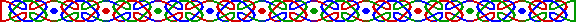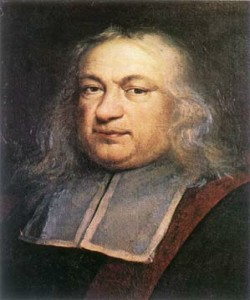(照片取自「The MacTutor History of Mathematics Achieve」http://www-gap.dcs.st-and.ac.uk/~history/ )1640年 6月，法國數學大師費馬 (Pierre de Fermat 1601-1665) 在給梅森 (Marin Mersenne 1588-1648) 的信中提及若干事實，詳見《梅森素數》一文，其中：

ap-1 = 1 (mod p)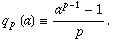qp(ab) = qp(a) + qp(b)

qp(p+1) = -1；qp(p-1) = 1底數 n 偽素數 斯洛恩數列 2 341、561、645、1105、1387、1729、1905、2047、2465、2701、2821、3277、4033、4369、4371、4681、... A001567 3 91、121、286、671、703、949、1105、1541、1729、1891、2465、2665、2701、2821、3281、3367、3751、4961、... A005935 2 & 3 1105、1729、2465、2701、2821、... A052155

 底 最小偽素數 2 341 = 11 * 31 3 91 = 7 * 13 5 217 = 7 * 31 7 25 = 5 * 5 2, 3 1105 = 5 * 13 * 17 2, 5 561 = 3 * 11 *17 2, 7 561 = 3 * 11 *17 3, 5 1541 = 23 * 67 3, 7 703 = 19 * 73 5, 7 561 = 3 * 11 * 17 2, 3, 5 1729 = 7 * 13 * 19 2, 3, 7 1105 = 5 * 13 * 17 2, 5, 7 561 = 3 * 11 * 17 3, 5, 7 29341 = 13 * 37 * 61 2, 3, 5, 7 29341 = 13 * 37 * 61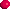n 不能被一完全平方數整除。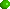n 是奇數，且至少含有三個不同的素因子。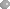對於 n 的每一個素因子 p ，n-1 能被 p-1 整除。

8911 = 7*19*67，顯然滿足了首兩個條件。

8911-1 = 8910 = 2*32*5*11

7-1 = 6 = 2*3、19-1 = 18 = 2*32、67-1 = 66 = 2*3*11 全是 8910 的因子。

1939年，卓域克 (J. Chernick) 給出了一個建構卡邁克爾數的方法：

M3(m) = (6m + 1) (12m + 1) (18m + 1) ，若式中三個因子皆為素數，這 M3(m) 便是卡邁克爾數了。

M4(m) = (6m + 1) (12m + 1) (18m + 1) (36m + 1)

M5(m) = (6m + 1) (12m + 1) (18m + 1) (36m + 1) (72m + 1)Ball, W. W. R. and Coxeter, H. S. M. Mathematical Recreations and Essays, 13th ed. New York: Dover, p. 61, 1987.

Beiler, A. H. Recreations in the Theory of Numbers: The Queen of Mathematics Entertains. New York: Dover, 1966.

Carmichael, R. D. "Note on a New Number Theory Function." Bull. Amer. Math. Soc. 16, 232-238, 1910.

Conway, J. H. and Guy, R. K. The Book of Numbers. New York: Springer-Verlag, pp. 141-142, 1996.

Guy, R. K. "Carmichael Numbers." §A13 in Unsolved Problems in Number Theory, 2nd ed. New York: Springer-Verlag, pp. 30-32, 1994.

Guy, R. K. "Pseudoprimes. Euler Pseudoprimes. Strong Pseudoprimes." §A12 in Unsolved Problems in Number Theory, 2nd ed. New York: Springer-Verlag, pp. 27-30, 1994.

Ribenboim, P. "The Little Book of Bigger Prime" , New York: Springer-Verlag, 1991

Weisstein, E. W. "Carmichael Number." From MathWorld. http://mathworld.wolfram.com/CarmichaelNumber.html.

Weisstein, E. W. "Fermat's Little Theorem." From MathWorld. http://mathworld.wolfram.com/FermatsLittleTheorem.html.

Weisstein, E. W. "Pseudoprime." From MathWorld. http://mathworld.wolfram.com/Pseudoprime.html.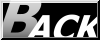Hosted by www.Geocities.ws